# Luna Tech

Tutorials For Dummies.

# 0. 前言

Reference: 8.15-8.16

# 1. General Depth First Search

## General DFS 难还是特殊 DFS 难？

General 的 DFS 比特殊的 DFS 要容易一些，因为它的目标是尽可能深入地找、尽可能连接更多的node，需要的时候就分叉：

• search as deeply as possible,
• connecting as many nodes in the graph as possible and
• branching where necessary.

## 如何构造 search tree？

DFS 和 BFS 一样，也会利用 predecessor 的信息来构造 tree。

DFS 的 Node 还需要额外的两个变量，一个是 discovery time，一个是 finish time。

Discovery time 用来记录这个 node 第一次被访问时我们一共走了多少步。

Finish time 就是一个 node 被标记为黑色（访问结束）之前我们一共走了多少步。

# 2. General DFS Code

• Inherit from Graph class

• dfs method 会遍历所有的 node，当一个 node 是白色（未被访问过）时，call dfsvisit method。

这么做（遍历而不是直接从 start node 开始 search）的原因是：我们要确保所有 graph 里面的 node 都查看过了，在构造 depth first forest 时不遗漏任何一条路径。

• dfsvisit 会 explore 所有白色的neighbour node，做深度搜索。

这个 method 跟 bfs 基本是一样的，唯一的区别就是 for loop 里面最后一行的 recursive self.dfsvisit(nextVertex)

bfs 在这里是把 node 加入到了 queue 里面，稍后再搜索。

所以 bfs 用的是 queue，dfs 用的是 stack (recursive call)

# pip3 install pythonds
from pythonds.graphs import Graph
class DFSGraph(Graph):
def __init__(self):
super().__init__() # inherit from Graph
self.time = 0 # extra field

# extra method
def dfs(self):
for aVertex in self:
aVertex.setColor('white')
aVertex.setPred(-1)
for aVertex in self:
if aVertex.getColor() == 'white':
self.dfsvisit(aVertex)

# extra method, helper
def dfsvisit(self,startVertex):
startVertex.setColor('gray')
self.time += 1
startVertex.setDiscovery(self.time)
for nextVertex in startVertex.getConnections():
if nextVertex.getColor() == 'white':
nextVertex.setPred(startVertex)
self.dfsvisit(nextVertex)
startVertex.setColor('black')
self.time += 1
startVertex.setFinish(self.time)


# 3. 图解 DFS 步骤

## 1. 构造 DFS tree，从 A 开始，A 变灰色

A 的 Discovery time = 1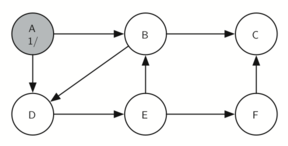## 2. A 到 B

A 有两个 child，B 和 D，我们根据字母顺序选 - B

B 的 Discovery time = 2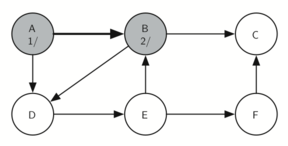## 3. B 到 C （继续深入）

B 有两个 child，C 和 D，我们根据字母顺序选 - C

C 的 Discovery time = 3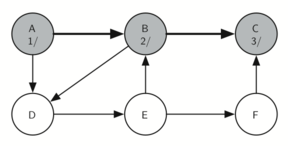## 4. C 没有 child，所以变成黑色（访问结束）

C 是这个 branch 里面的最后一个 node，所以C 的 finish time = 4（注意这里即使没有访问新的 node，也要在 Discovery time 上 + 1）。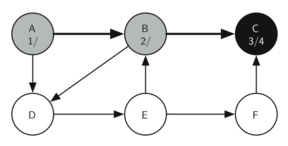## 5. 回到 B，访问它的另一个 child - D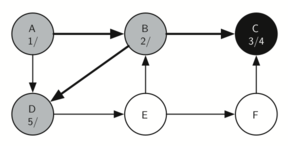## 6. 继续访问 D 的 child - E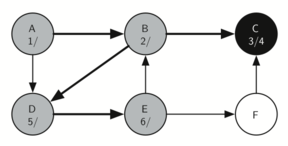## 7. 继续访问 E 的 child - F

E 有两个 child - B 和 F，因为 B 已经是灰色了，所以我们不能继续访问 B（会陷入死循环）。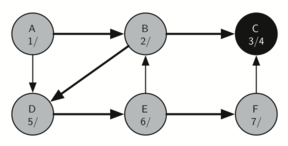## 8. F 的 child 是 C，C 是黑色，所以 F 开始返回起点

F 也被标记成黑色，这个 branch 访问结束。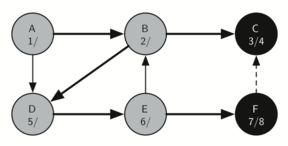## 9. 从 F 返回 E，E 的两个 child 都被访问过，所以 E 变黑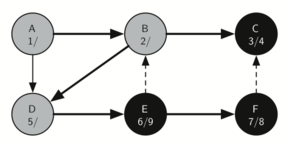## 10. 从 E 返回 D，D 变黑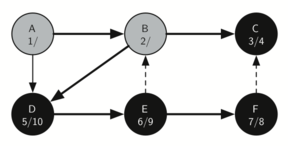## 11. 从 D 返回 B，B 变黑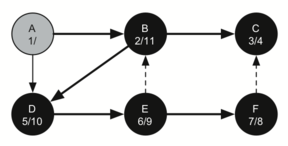## 12. 从 B 回到 A，整条路径完成

A - B - D - E - F - C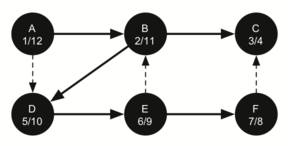## parenthesis property 括号属性

• 比 parent 长的 discovery time
• 比 parent 短的 finish time

The starting and finishing times for each node display a property called the parenthesis property. This property means that all the children of a particular node in the depth first tree have a later discovery time and an earlier finish time than their parent.

Although our implementation of bfs was only interested in considering nodes for which there was a path leading back to the start, it is possible to create a breadth first forest that represents the shortest path between all pairs of nodes in the graph.

# 4. DFS 效率分析

## Main Method

def dfs(self):
for aVertex in self:
aVertex.setColor('white')
aVertex.setPred(-1)
for aVertex in self:
if aVertex.getColor() == 'white':
self.dfsvisit(aVertex)


## Helper Method

def dfsvisit(self,startVertex):
startVertex.setColor('gray')
self.time += 1
startVertex.setDiscovery(self.time)
for nextVertex in startVertex.getConnections():
if nextVertex.getColor() == 'white':
nextVertex.setPred(startVertex)
self.dfsvisit(nextVertex)
startVertex.setColor('black')
self.time += 1
startVertex.setFinish(self.time)


helper method 的时间复杂度等于一个 node 的 edge 数量，就是 O(m)。